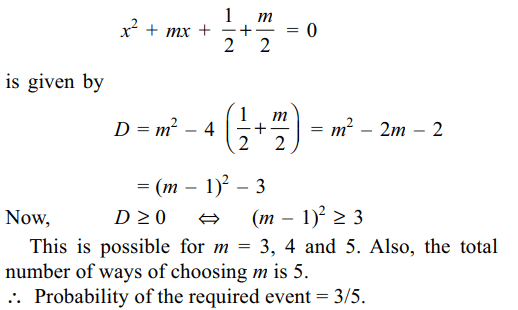## Probability Questions and Answers Part-2

1. One hundred identical coins, each with probability p of showing heads are tossed once. If 0 < p < 1 and the probability of heads showing on 50 coins is equal to that of heads showing on 51 coins, the value of p is
a) 1/2
b) 51/101
c) 49/101
d) 3/101

Explanation: Let X be the number of coins showing heads. Then X follows a binomial distribution with parameters n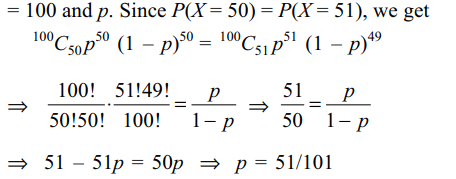2. Suppose X follows a binomial distribution with parameters n and p, where 0 < p < 1.If P(X = r)/P(X = n – r) is independent of n for every value of r, then
a) p = 1/2
b) p = 1/3
c) p = 1/4
d) p = 1/5

Explanation: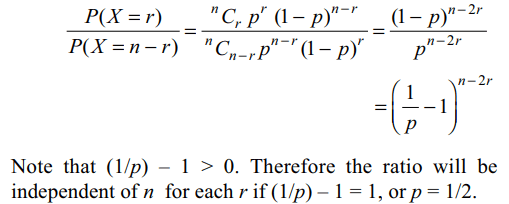3. The minimum number of times a fair coin must be tossed so that the probability of getting at least one head is at least 0.8 is
a) 7
b) 6
c) 5
d) 3

Explanation: Suppose the coin is tossed n times. Let X be the number of heads obtained. Then X follows a binomial distribution with parameters n and p = 1/2. We have P(X≥ 1) ≥ 0.8 $\Rightarrow$ 1 – P(X = 0) ≥ 0.8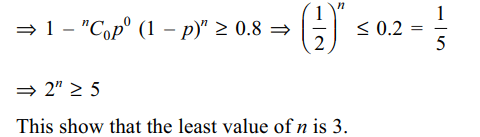4. For the three events A, B and C, P (exactly one of the events A or B occurs) = P(exactly one of the events B or C occurs) = P(exactly one of the events C or A occurs) = p and P(all the three events occur simultaneously) = $p^{2}$, where 0 < p < 1/2. Then the probability of at least one of the three events A, B and C occurring is
a) $\frac{3p+2p^{2}}{2}$
b) $\frac{p+3p^{2}}{4}$
c) $\frac{p+3p^{2}}{2}$
d) $\frac{3p+2p^{2}}{4}$

Explanation: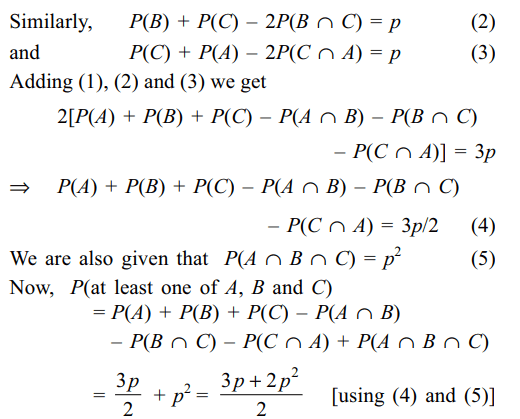5. Nine identical balls are numbers 1, 2, ...9. are put in a bag. A draws a ball and gets the number a. The ball is put back the bag. Next B draws a ball gets the number b. The probability that a and b satisfics the inequality a – 2b + 10 > 0 is
a) $\frac{52}{81}$
b) $\frac{55}{81}$
c) $\frac{61}{81}$
d) $\frac{62}{81}$

Explanation: a – 2b + 10 > 06. An unbiased die with faces marked 1, 2, 3, 4, 5 and 6 is rolled four times. Out of four face values obtained, the probability that the minimum face value is not less than 2 and the maximum face value is not greater than 5 is
a) 16/81
b) 1/81
c) 80/81
d) 65/81

Explanation:7. If the papers of 4 students can be checked by any one of the 7 teachers, then the probability that all the 4 papers are checked by exactly 2 teachers is
a) 2/7
b) 6/49
c) 32/343
d) 1/7

Explanation: The total number of ways in which papers of 4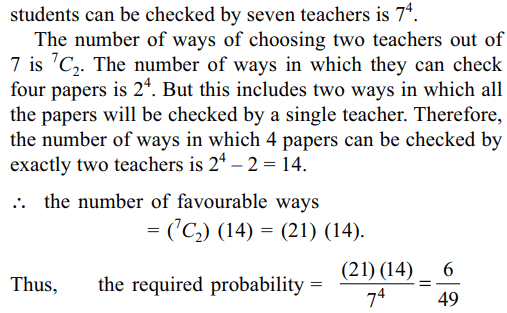8. Let A, B,C, be three mutually independent events. Consider the two statements $S_{1}$ and $S_{2}$
$S_{1} : A$  and B $\cup C$  are independent
$S_{2} : A$  and B $\cap C$  are independent
Then
a) Both $S_{1}$ and $S_{2}$ are true
b) Only $S_{1}$ is true
c) Only S2 is true
d) Neither $S_{1}$ nor $S_{2}$ is true

Explanation:9. If m is a natural such that $m \leq 5$  , then the probability that the quadratic equation $x^{2}+mx+\frac{1}{2} +\frac{m}{2}=0$       has real roots is
a) 1/5
b) 2/3
c) 3/5
d) 1/5